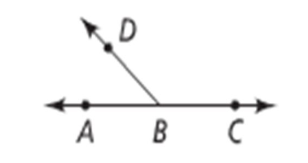QUESTION

# If two adjacent angles are supplementary, they form a ______.

Hint: Here, we will consider an example to find the relation of adjacent angles.Supplementary angles are those whose sum measures ${180^ \circ }$ .If you look at the above figure, $\angle ABC + \angle DBC = {180^ \circ }$.
Therefore, $\angle ABC\& \angle DBC$ form a linear pair. Since, we know that the sum of angles in linear pair is ${180^ \circ }$
Note: Before solving the given problem make sure you go through the basics of the Lines and Angles. Supplementary angles are those whose sum measures ${180^ \circ }$ and they form a linear pair and Complement angles are those whose sum measures ${90^ \circ }$ and they form a right angle triangle. Make sure you do not take the values wrongly.